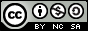- 目录 -
HihoCoder - 1044 状态压缩一 (状压dp)

## 样例输入

5 2 1
36 9 80 69 85


## 样例输出

201


## 思路

• $dp[i][j]$ 表示前 $i$ 个位置，选择的状态为 $j$ 时所能达到的最大价值。
• $j$ 表示的状态是，以第 $i$ 个位置为结尾的连续 $m$ 个位置的选取状态。

0 0 0
0 0 1
0 1 0
0 1 1
1 0 0
1 0 1
1 1 0
1 1 1


1 0 1 中最后一个 1 代表 4 这个位置已经被选择，那么 2 3 这两个位置的选择情况是 1 0 ，那么 2 3 这两个位置的状态不能改变，唯一能改变的就是 1 这个位置的状态，那么我们就有 dp[0 1 0]dp[1 1 0]这两个状态可以转移到 dp[1 0 1]

• 当前状态的最后一位为 1 时（选择这个位置）：

$dp[i][j] = max(dp[i - 1][j » 1], dp[i - 1][(j » 1) | (1 « (m - 1))]) + w[i]​$

• 当前状态的最后一位为 0 时（不选这个位置）：

$dp[i][j] = max(dp[i - 1][j » 1], dp[i - 1][(j » 1) | (1 « (m - 1))])$

## 代码

  1 2 3 4 5 6 7 8 9 10 11 12 13 14 15 16 17 18 19 20 21 22 23 24 25 26 27 28 29 30 31 32 33 34 35 36 37 38 39 40 41  #include using namespace std; #define mem(a, b) memset(a, b, sizeof(a)) const int N = 1e3 + 10; int dp[N][(1 << 10) + 5]; int w[N]; int get_cnt(int n) { int cnt = 0; while (n) { cnt++; n = n & (n - 1); } return cnt; } int main() { //freopen("in.txt", "r", stdin); int n, m, q; scanf("%d%d%d", &n, &m, &q); for (int i = 1; i <= n; i++) scanf("%d", &w[i]); for (int i = 1; i <= n; i++) { for (int j = 0; j < (1 << m); j++) { if (get_cnt(j) > q) continue; if (j & 1) dp[i][j] = max(dp[i - 1][j >> 1], dp[i - 1][(j >> 1) | (1 << (m - 1))]) + w[i]; else dp[i][j] = max(dp[i - 1][j >> 1], dp[i - 1][(j >> 1) | (1 << (m - 1))]); } } int ans = 0; for (int i = 0; i < (1 << m); i++) ans = max(ans, dp[n][i]); printf("%d\n", ans); return 0; }